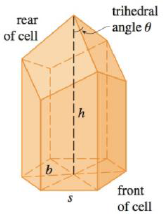Chapter 4.7, Problem 47E### Single Variable Calculus: Early Tr...

8th Edition
James Stewart
ISBN: 9781305270343

#### Solutions

Chapter
Section### Single Variable Calculus: Early Tr...

8th Edition
James Stewart
ISBN: 9781305270343
Textbook Problem

# In a beehive, each cell is a regular hexagonal prism, open at one end with a trihedral angle at the other end as in the figure. It is believed that bees form their cells in such a way as to minimize the surface area for a given side length and height, thus using the least amount of wax in cell construction. Examination of these cells has shown that the measure of the apex angle θ is amazingly consistent. Based on the geometry of the cell, it can be shown that the surface area S is given by S = 6 s h − 3 2 s 2 cot θ + ( 3 s 2 3 / 2 ) csc θ where s, the length of the sides of the hexagon, and h, the height, are constants.(a) Calculate dS/dθ.(b) What angle should the bees prefer?(c) Determine the minimum surface area of the cell (in terms of s and h).Note: Actual measurements of the angle θ in beehives have been made, and the measures of these angles seldom have been made, and the measures of these angles seldom differ from the calculated value by more than 2°.(a)

To determine

To calculate: dSdθ

Explanation

Given:

The surface area of regular hexagonal prison is,

S=6sh32s2cot(θ)+(3s232)csc(θ)

Where, S is the length of the sides of the hexagon, and h is the height are constants.

Calculation:

Differentiate S with respect to θ,

dSdθ=32s2csc2(θ)3

(b)

To determine

To find: The angle that the bees prefer.

(c)

To determine

To find: The minimum surface area of the cell.

### Still sussing out bartleby?

Check out a sample textbook solution.

See a sample solution

#### The Solution to Your Study Problems

Bartleby provides explanations to thousands of textbook problems written by our experts, many with advanced degrees!

Get Started

#### 23. If and , find (a) (b) (c) (d)

Mathematical Applications for the Management, Life, and Social Sciences

#### Simplify: 915

Elementary Technical Mathematics

#### An integral for the solid obtained by rotating the region at the right about the y-axis is:

Study Guide for Stewart's Single Variable Calculus: Early Transcendentals, 8th